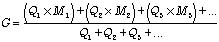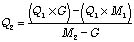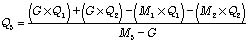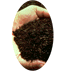## Moisture Content

### by Nancy Trautmann and Tom Richard

When deciding what proportions of various materials to mix together in making compost, the moisture of the resulting mixture is one of the critical factors to consider. The following steps outline how to design your intital mix so that it will have a suitable moisture level for optimal composting. In the composting industry, the convention is to report moisture content on a wet (or total weight) basis, as the formulas below indicate.

1. Calculate the % moisture for each of the materials you plan to compost.
a) Weigh a small container.
b) Weigh 10 g of the material into the container.
c) Dry the sample for 24 hours in a 105-110 degree C oven.
d) Reweigh the sample, subtract the weight of the container, and determine the moisture content using the following equation:

Mn = ((Ww-Wd)/Ww) x 100
in which:
Mn = moisture content (%) of material n
WW = wet weight of the sample, and
Wd = weight of the sample after drying.

Suppose, for example, that you weigh 10 g of grass clippings (Ww) into a 4 g container and that after drying the container plus clippings weighs 6.3 g. Subtracting out the 4-g. container weight leaves 2.3 g as the dry weight (Wd) of your sample. Percent moisture would be:

Mn = ((Ww-Wd)/Ww) x 100
= ((10 - 2.3) / 10) x 100
= 77% for the grass clippings

2. Choose a moisture goal for your compost mixture. Most literature recommends a moisture content of 50%-60% by weight for optimal composting conditions.
3. The next step is to calculate the relative amounts of materials you should combine to achieve your moisture goal. The general formula for percent moisture is:in which:
Qn = mass of material n ("as is", or "wet weight")
G = moisture goal (%)
Mn = moisture content (%) of material n

You can use this formula directly to calculate the moisture content of a mixture of materials, and try different combinations until you get results in a reasonable range. If you have a browser capable of handling Java script, you can try this formula out for up to 3 materials. (Calculate Percent Moisture of Compost)

Using trial and error to determine what proportions to use for a mixture will work, but there is a faster way. For two materials, the general equation can be simplified and solved for the mass of a second material (Q2) required in order to balance a given mass of the first material (Q1). Note that the moisture goal must be between the moisture contents of the two materials being mixed.For example, suppose you wish to compost 10 kg grass clippings (moisture content = 77%). In order to achieve your moisture goal of 60% for the compost mix, you calculate the mass of leaves needed (moisture content = 35%):Q2= ((10 kg)(60) - (10 kg)(77)) / (35 - 60)
= 6.8 kg leaves

Mixtures of 3 or more materials can also be solved in a similar way (although the algebra is more complicated), but for an exact solution the amounts of all but one material must be specified. To find the mass of the third material (Q3) given the masses of the first two (Q1 and Q2) plus all three moisture contents (M1, M2, and M3) and a goal (G), solve:With an internet browser that incorporates the JavaScript language, you can try calculating mixtures ratios based on moisture goals for up to three materials. (Calculate Required Weight of Third Ingredient for Ideal Moisture Content)Cornell Composting Science & Engineering Composting in Schools Resources